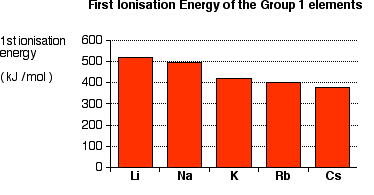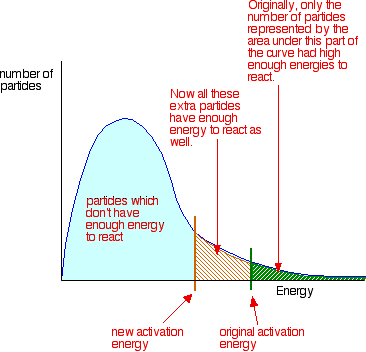# What reaction takes place between Cesium and water that makes it react so violently?

Dec 23, 2014

Cesium reacts with cold water to form hydrogen gas and a solution of cesium ions and hydroxide ions.

2Cs(s) + 2H₂O(l) → H₂(g) + 2Cs⁺(aq) + 2OH⁻(aq)

The reaction is so explosive that it often shatters the container.

We can write the changes for the Cs atom as

(1) Cs(s) → Cs(g); ΔH_"sub" = +79 kJ/mol
(2) Cs(g) → Cs⁺(g); ${I}_{1}$ = +375.7 kJ/mol
(3) Cs⁺(g) → Cs⁺(aq); ΔH_"hydr" = -276 kJ/mol

The sublimation energy (1) is the smallest of the alkali metals because the Cs atoms are the biggest.

As the atoms get bigger, the strengths of the metallic bonds decrease. It takes less energy to get the atoms into the vapour phase.

The ionization energy (2) is the smallest of the alkali metals.

As you go down the Group, the valence electron is further from the nucleus and is screened by more layers of inner electrons.The energy input steps (1) and (2) are related to the activation energy of the reaction. So the activation energy for Cs is the lowest, mainly because of its low ionization energy.

The lower the activation energy, the faster the reaction. So Cs reacts extremely fast — and you get an explosion.Here's a video showing the reaction of Cs with water.